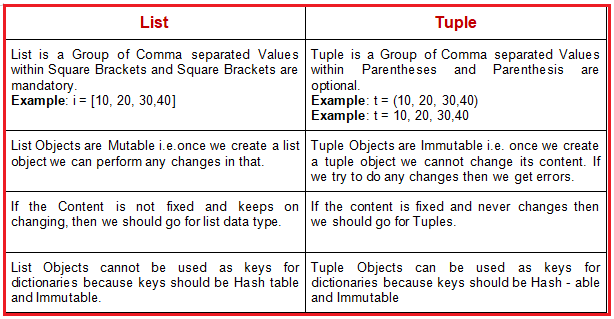# Tuples in Python

## Tuples in Python with Examples

In this article, I am going to discuss Tuples in Python with examples. Please read our previous article where we discussed Lists in Pythonwith examples. As part of this article, we are going to discuss the following pointers which are related to Tuples in Python.

1. What is tuple data structure?
2. Different ways to create a tuple.
3. Accessing elements of tuple
4. Tuple vs immutability
5. Important functions and methods of Tuple:
6. Tuple packing
7. Tuple unpacking:
8. Tuple comprehensions
9. Differences between List and Tuple
10. Multiple Programs to understand above concepts
##### What are Tuples in Python?

A tuple refers to a collection of objects in python which are ordered and can’t be changed. The python objects here may be strings, lists, tuples, integers etc.

##### Characteristics and Features of Tuples in Python:
1. Insertion order is preserved: The order in which elements are added to the tuple is the order in which the output will be displayed.
2. Immutable: Once if we create a tuple object, then we cannot modify the content of the tuple object. This is the only feature which differentiates tuples from the lists.
3. Tuple can store duplicates.
4. Tuple can store the same type of objects and different types of objects as well.
5. Index plays the main role in tuple.
6. Tuple supports both positive and negative indexes. Positive index means forward direction (from left to right). Negative index means backward direction (from right to left).
7. We can create a tuple by using parenthesis () symbol. Parenthesis is optional but it’s recommended to use.
8. Inside the tuple every object should be separated by comma as a separator.
##### When should we go for tuple data structure in python?

If we are going to define a data which is not going to change over the period, then we should go for tuple data structure. For example, weekdays, calendar months, years etc. Let us see an example for better understanding.

###### Example: Tuple having same type of objects (demo1.py)
```employee_ids = (1, 2, 3, 4, 5)
print("same type of objects:",employee_ids)
print(type(employee_ids))```
###### Output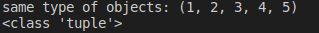Note: parenthesis are optional, when creating tuples.

###### Example: Parenthesis is optional for tuple (Demo2.py)
```employee_ids = 1, 2, 3, 4, 5
print("same type of objects:",employee_ids)
print(type(employee_ids))```
###### Output###### Example: Different elements in tuple (demo3.py)
```employee_details =  (1, "RGV", 1000.123)
print("different type of objects:", employee_details)```

Output: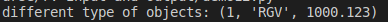##### Single Value tuple

If a tuple has only one object, then that object should end with a comma separator otherwise python, internally, will not consider it as a tuple.

###### Example: Single Value Tuple (demo4.py)
```name =("Sushanth")
print(name)
print(type(name))```
###### Output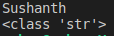###### Example: Single Tuple value (demo5.py)
```a = (11)
print(a)
print(type(a))```

Output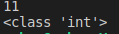###### Example: Single Tuple (demo6.py)
```name =("Sushanth",)
print(name)
print(type(name))```
###### Output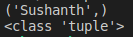##### Different Ways to Create Tuples in Python:
1. Empty Tuple
2. Single value tuple
3. Tuple with group of values
4. By using tuple() function
##### Empty Tuple in Python:

We can create an empty tuple by using empty parenthesis.

###### Example: Empty Tuple (demo7.py)
```emp_id = ()
print(emp_id)
print(type(emp_id))```
###### Output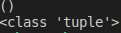##### Single Value Tuple

We can create a single value tuple in two ways:

1. Using parenthesis – Comma is mandatory.
2. Without using parenthesis – Comma is mandatory
###### Example:Single Value Tuple (demo8.py)
```emp_id = (11,)
std_id = 120,
print(emp_id)
print(std_id)```
###### Output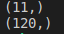##### Tuple with group of values

Tuple can contain group of objects; those objects can be of the same type or different types. While creating tuple with group of values parentheses are optional.

###### Example: Tuple with group of Values (demo9.py)
```emp_id = (11, 12, 13)
std_id = 120, 130, 140
print(emp_id)
print(std_id)```
###### Output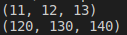##### By using tuple() function

We can create a tuple by using tuple() function.

###### Example: Using Tuple Function (demo10.py)
```l = [11, 22, 33]
t=tuple(l)
print(t)```

Output: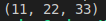###### Example: Using Tuple Function (demo11.py)
```t=tuple(range(1, 10, 2))
print(t)```

Output: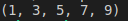##### Different Ways to Access Tuples in Python:

We can access tuple elements in three ways,

1. Indexing
2. Slicing operator
3. Looping

These are the methods which we discussed in the lists chapter. So, let’s move on with examples.

##### Accessing Tuples using Indexing in Python:

Index refers to the position where the element is stored. This is same as what we discussed in case of strings and lists.

###### Example: Indexing (demo12.py)
```t=(10,20,30,40,50,60)
print(t)           #   10 #Positive indexing
print(t[-1])          #   60 #Negative indexing
#print(t)        #   IndexError: tuple index out of range```
###### Output: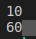##### Accessing Tuples using Slicing operator in Python:

Example: Slicing Operator(demo13.py)

```t=(10,20,30,40,50,60)
print(t[2:5])
print(t[2:100])
print(t[::2])```
###### Output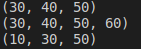##### Accessing Tuples using Looping in Python:

Example: Looping(demo14.py)

```t=(10,20,30,40,50,60)
for i in t:
print(i)```
###### Output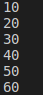##### TUPLE IMMUTABILITY

Tuple having immutable nature. If we create a tuple then we cannot modify the elements of the existing tuple. The operations/functions/methods on tuples, which we are going to see now, will create and return new objects (lists, strings, tuples) depending on the operation, but not change the existing tuple because of its immutability.

###### Example: Immutability (demo15.py)
```t=(10,20,30,40)
t=70```

Output: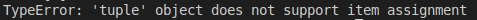##### MATHEMATICAL OPERATORS

We can apply plus (+) and Multiplication (*) operators on tuple.

1. + operator works as concatenation.
2. * operator works as multiplication.
##### Concatenation operator (+):

This operator concatenates two tuples and returns a single new tuple object.

###### Example: Concatenation (demo16.py)
```t1=(10,20,30)
t2=(40,50,60)
t3=t1+t2
print(t3)```

Output: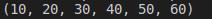##### Multiplication operator (*):

Multiplication operator works as repetition operator, and returns a single, new, repeated tuple object.

###### Example: Multiplication (demo17.py)
```t1=(10,20,30)
t2=t1*3
print(t2)```

Output: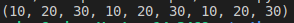##### IMPORTANT METHODS and FUNCTIONS of Tuples in Python:
1. len() function
2. count() method
3. index() method
4. sorted() function
5. min() and max() functions
##### len() function in Tuples:

It returns number of elements present in the tuple.

###### Example: len() function (demo18.py)
```t=(10,20,30,40)
print(len(t))```

Output: 4

##### count() method in Tuples:

It returns number of occurrences of given element in the tuple.

###### Example: count() method (demo19.py)
```t=(10,20,10,10,20)
print(t.count(10))```

Output: 3

##### index() method in Tuples:

It returns the index of first occurrence of the given element. If the specified element is not available, then we will get ValueError.

###### Example: index() method (demo20.py)
```t=(10,20,10,10,20)
print(t.index(10))
# print(t.index(30))#     #  ValueError```

Output: 0

###### Example: index() method in Tuples (demo21.py)
```t=(10,20,10,10,20)
#print(t.index(10))
print(t.index(30))#     #  ValueError```

Output: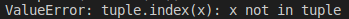##### sorted() function in Tuples:

This function can sort the elements which is default natural sorting order. This returns a sorted list of elements.

###### Example: sorted() method in Tuples (demo22.py)
```t=(40,10,30,20)
t1=sorted(t)
print(t)
print(t1)```
###### Output: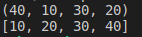We can sort according to reverse of default natural sorting order as below

###### Example: sorted() method in Tuples (demo23.py)
```t=(40,10,30,20)
t1=sorted(t,reverse=True)
print(t1)```

Output: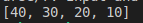##### min() and max() functions in Tuples:

These functions return min and max values according to default natural sorting order.

###### Example: min() and max() method in Tuples (demo24.py)
```t=(40,10,30,20)
print(min(t))    # 10
print(max(t))    # 40```
###### Output: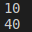##### TUPLE PACKING

We can create a tuple by packing a group of variables.

###### Example: tuple packing (demo25.py)
```a=10
b=20
c=30
d=40
t=a, b, c, d
print(t)```

Output: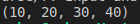##### TUPLE UNPACKING

Tuple unpacking is the reverse process of tuple packing, we can unpack a tuple and assign its values to different variables

###### Example: tuple unpacking (demo26.py)
```t=(10, 20, 30, 40)
a, b, c, d = t
print("a=",a , "b=" , b," c=", c ,"d=",d)```

Output: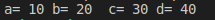Note: At the time of tuple unpacking the number of variables and number of Values should be the same, otherwise we will get ValueError.

##### TUPLE COMPREHENSION

Tuple comprehension is not supported by Python. For example, let’s consider,

t= (x**2 for x in range (1,6))

This expression after execution will return a generator object in ‘t’ rather than a tuple object. We need to run it using a loop

###### Example: Tuple comprehension (demo27.py)
```t= ( x**2 for x in range(1,6))
print(type(t))
for x in t:
print(x)```
###### Output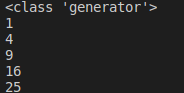##### DIFFERENCES BETWEEN LISTS and TUPLES in PYTHON:

List and Tuple are exactly the same except for a small difference: List objects are mutable Whereas Tuple objects are immutable. In both cases insertion, the order is preserved, duplicate objects are allowed, heterogeneous objects are allowed, index and slicing are supported.